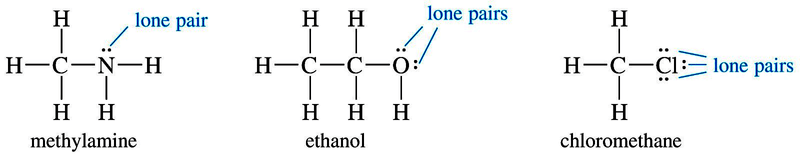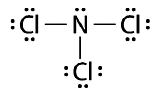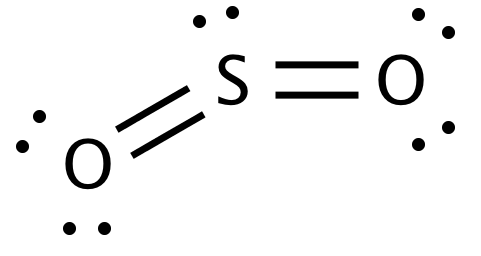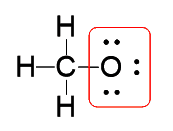•Sign In
• Blog
• Write for UsUh Oh! It seems you’re using an Ad blocker!

We always struggled to serve you with the best online calculations, thus, there's a humble request to either disable the AD blocker or go with premium plans to use the AD-Free version for calculators.

Or# Formal Charge Calculator

Enter the number of valence electrons, number of lone and bonded pairs. The calculator will readily determine the formal charge.

No. of Valence Electrons:

No. of Lone Pair Electrons:

No. of Bound Electrons:

Table of Content

 1 What is priceeight Class? 2 priceeight Class Chart: 3 How to Calculate priceeight Density (Step by Step): 4 Factors that Determine priceeight Classification: 5 What is the purpose of priceeight Class? 6 Are mentioned priceeight Classes verified by the officials? 7 Are priceeight Classes of UPS and FedEx same?

Get the Widget!

Add this calculator to your site and lets users to perform easy calculations.

Feedback

How easy was it to use our calculator? Did you face any problem, tell us!

An online formal charge calculator is exclusively designed to calculate formal charge of an atom. Moreover, it should be kept in mind that we must have a sound knowledge of the formal charge chemistry before you start using this free calculator. So, without getting late, let’s dive in!

## What Is A Formal Charge?

A formal charge is defined as:

“The individual charge of each atom present in a molecule”

Determining the formal charge is of great significance. This is because it shows how reactive a molecule is and how it will behave while creating bonds with either atoms.

### Lewis Structure:

This particular structure displays the bonding electron pairs of atoms in a molecule. Also, the presence of lone pairs in the molecules is also shown using this pattern.

In Lewis Structure:

• A single bond is formed by two electrons and is denoted by “:” or “-”
• A double bond is create with 4 electrons and is shown as “::” or “=”
• A triple bond is formed using 6 shared electrons and is displayed as “:::” or “≡”
• The lone pair of electrons is shown as “:” always

No matter how complex the dot structure of a molecule is, the free online formal charge calculator assists you in calculating charge in a fragment of seconds.

#### Examples:

The Lewis structures of carbon dioxide is below:Similarly, the Lewis structure for sulphur dichloride is as follows:#### Lone Pair Electrons:

“A specific extra pair of electrons that does not take part in the bonding scheme is known as the lone pair”.

In practice, lone pairs of electrons are not usually shown. However, it is mandatory to remember them as well.#### Bonding Pair Electrons:

“The electron pairs that do take part in the bond formation are known as the bonding pair of electrons”.

For example:

The bond pair of electrons is Nitrogen Trichloride molecule are represented as “-” and lone pair as “:”### Formal Charge Formula:

You can calculate the formal charge of any atom with the help of the equation below:

$$FC = V – \left(LP + 0.5BE\right)$$

Where:

FC = Formal Charge on Atom
V = Number of Valence Electrons
LP = Lone Pair Electrons
BE = Number of Bonded Electrons

### How To Calculate Formal Charge?

Here we will be solving a couple of examples to make your concept even more stronger than before. Let’s start!

Example # 01:

We are seeking to calculate the formal charge of sulphur in sulphur dioxide molecule. How to find formal charge from Lewis structure?

Solution:

The lewis structure of the sulphur dioxide containing all the bonding and lone pair electrons is as follows:From the above structure, we have:

Valence shell electrons of sulphur = 6
Lone pair electrons of sulphur = 2
Bonded electrons of sulphur = 8

Using formal charge equation:

$$FC = V – \left(LP + 0.5BE\right)$$
$$FC = 6 – \left(2 + 0.5*8\right)$$
$$FC = 6 – \left(2 + 4\right)$$
$$FC = 6 – 6$$
$$FC = 0$$

Example # 02:

How to determine formal charge on fluorine?

Solution:

The dot structure of fluorine is as below:As we know that:

Valence shell electrons of sulphur = 7
Lone pair electrons of sulphur = 6
Bonded electrons of sulphur = 2

Calculating formal charge:

$$FC = V – \left(LP + 0.5BE\right)$$
$$FC = 7 – \left(6 + 0.5*2\right)$$
$$FC = 7 – \left(6 + 1\right)$$
$$FC = 7 – \left(7\right)$$
$$FC = 7 – 7$$
$$FC = 0$$

Except for the formula, our free online formal charge calculator takes a couple of seconds to generate accurate results.

Example # 03:

How to find formal charge on oxygen in $$CH_{3}O^-$$ ion?

Solution:

The lewis structure of $$CH_{3}O^-$$ is as follows:Carrying out formal charge calculation:

The number of bonding electrons oxygen in $$CH_{3}O^-$$ = 2
The number of valence shell electrons in $$CH_{3}O^-$$ = 6
Lone pair electrons = 6

Using formal charge formula:

$$FC = V – \left(LP + 0.5BE\right)$$
$$FC = 6 – \left(6 + 0.5*2\right)$$
$$FC = 6 – 7$$
$$FC = -1$$

### How Formal Charge Calculator Works?

Make a use of this free calculator to calculate formal charge on any atom contained within the molecule. Let’s find how!

Input:

• Enter the number of valence shell electrons
• Enter the number of the lone pair electrons the same way
• Finally, enter the value of the bonded electrons in the designated fields
• Tap the calculate button

Output:

The free formal charge calculator calculates:

• The exact formal charge for the respective atom in the molecule

## FAQ’s:

### What is the formal charge rule?

According to the formal charge rule, the number of the electrons shared among the atoms of the molecule must be in equilibrium. Moreover, this knowledge also provides an edge in determining the true Lewis structures.

### What is a negative formal charge?

When an atom donates more than a few electrons and there is an octet shell vacant in them, then there exists a possibility of gaining more electrons to fill the octet shell. This gain of electrons results in the negative formal charge on an atom.

### Are formal charges real charges?

No, the formal charges are not the real charges. They are just used to suppose the electrons transmission in between atoms for lewis structures.

### What is the best formal charge?

In practice, the formal charge of “0” is very best as it shows the atoms have their shells completely filled while bonding with each other in a molecule. Also, it is also known as the lowest formal charge.

### Does formal charge affect polarity?

No, the formal charge can never have any effect on the polarity. The formal charges are always assumed for the perfect covalent bonds which means there is no chance of electrons to be shared unequally. This cancels out any chance of polarity within the molecule.

### Does formal charge determine the dipole moment?

Yes, of course! The formal charge do determine the dipole moment

## Conclusion:

In chemical analysis, the formal charge is very crucial in predicting and establishing a reaction mechanism. As well, it helps you in keeping a proper track of the electron sharing among atoms. That is why using an online formal charge calculator help you in perfect formal charge calculations to avoid any hurdle during any chemical reaction.

From the source of Wikipedia: Chemical bond, Strong chemical bonds, Intermolecular bonding,

From the source of Khan Academy:  Types of Bonds, Ionic Bonds, Covalent Bonds, Polar covalent bonds, Comparison Of Covalent And Ionic Compounds